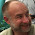## Friday, October 21, 2016

### The Metal in Two Beverage Cans

The Metal in Two Beverage Cans

Question:   One Coke can is 15 centimeters long.   The second coke can is 12 centimeters long.   Both coke cans hold 355 milliliters of liquid.

What is the radius of the top of each coke can?

What is the volume of the surface area of the two can?

What can is better for the environment?

Answer:  The procedure used to calculate the area of metal on the surface of a coke can given the height of the can and the volume of liquid in the cans is as follows.

Divide the volume of the can by the height of the can to get the area of the top or cross section of a circle parallel to the top of the can.   (This follows from the observation that the volume of the can is the product of the height and the circle around the can.)

Get the radius of the circle around the can by solving for r in the equation A=pi * r2.

Get the area of the top and bottom of the cans.  This is twice the area of the cross-section.

Get the area of the side of the can.   This is 2 x pi x r x height.

Add the area of the top, bottom and side.

Calculations are laid out below.

 Amount of Metal in Two Coke Cans Height of can in Centimeters 15 12 Volume of Can in Milliliters 355 355 Area of cross section of can in Centimeters 23.6667 29.5833 pi 3.1416 3.1416 Radius of cross-section (Area/pi)0.5  in centimeters 2.7447 3.0687 Area of Metal in Entire Can (Sum of volume of top and bottom and side)  Square Centimeters 306.0145 290.5381

Discussion of the environmental significance of this result:  The standard can is 12 centimeters long but I have on my desk a can of La Croix sparkling water that is 15 centimeters long.   The can with a 15-centimeter height has around 5.33% more metal in it than a can with a 12-centimeter height.

The typical empty soda can (one with a 12-centimeter height) weighs around 15 grams.  This means the can with a 15-centimeter height weighs around 15.8 grams.

According to one web site the world consumes around 200 billion aluminum beverage cans each year.   Let’s assume that 5.0 percent of cans are non-standard and weigh around 5.3% more than the standard.  I am admittedly uncertain about the validity of this assumption.

Based on this assumption I calculate tons of aluminum wasted by  assuming that 10 million cans deviate from the standard can by having 5.3% more aluminum.

The extra aluminum stemming from the deviation for the standard can is 7,990,188 grams or a bit over 8.8 tons of aluminum.  (There are 907,185 grams in one U.S. ton.)

So if you want to somewhat reduce the amount of aluminum used in the world and the amount of energy used to make this aluminum buy 355-milliliter (12 ounce) soft drinks in cans that are 12 millimeters high rather than soda in cans that is 15 millimeters high.

This leads to the question should government’s regulate the size of soda cans?  This question is beyond the scope of the current post.

Note to math teachers:  There are actually a lot more beverage can math problems that should be created.   You could have your students calculate the area of metal given the radius of the top.   You could have them use Excel or calculus to figure out the size of the can that uses the least amount of metal given the volume of liquid in the can.   More problems of this type will follow.

1.2.3.